# RS Aggarwal Solutions for Class 6 Chapter 3 Whole Numbers Exercise 3F

RS Aggarwal Solutions for Class 6, Chapter 3, Whole Numbers are given in PDF here. Practicing problems meticulously help the students score high marks in their exams. Exercise 3F of RS Aggarwal Solutions has the objective questions covering the entire chapter. Expert tutors prepared these solutions to help students in their exam preparation and also score high marks. Download RS Aggarwal Solutions for Class 6 in their respective links.

## Download PDF of RS Aggarwal Solutions for Class 6 Chapter 3 Whole Numbers Exercise 3F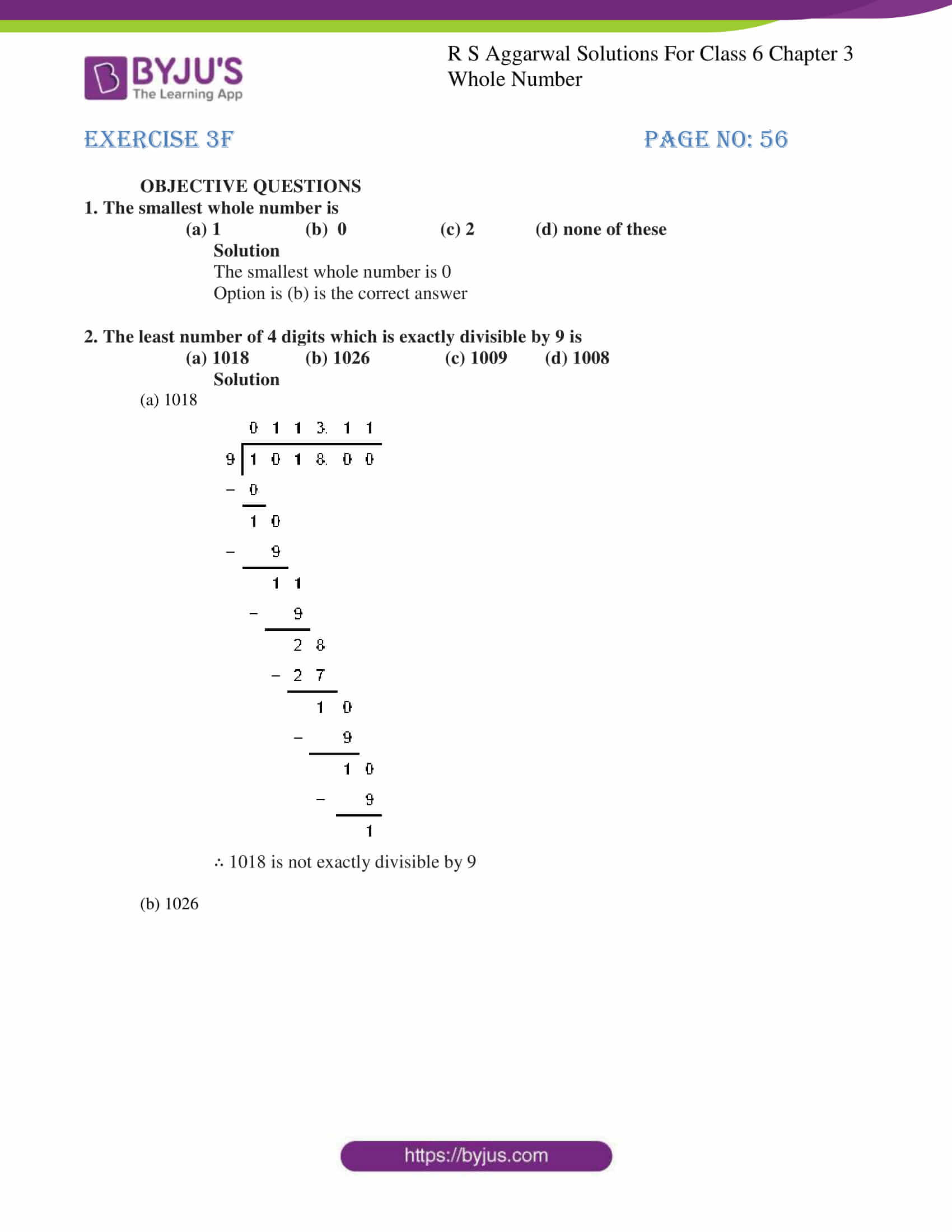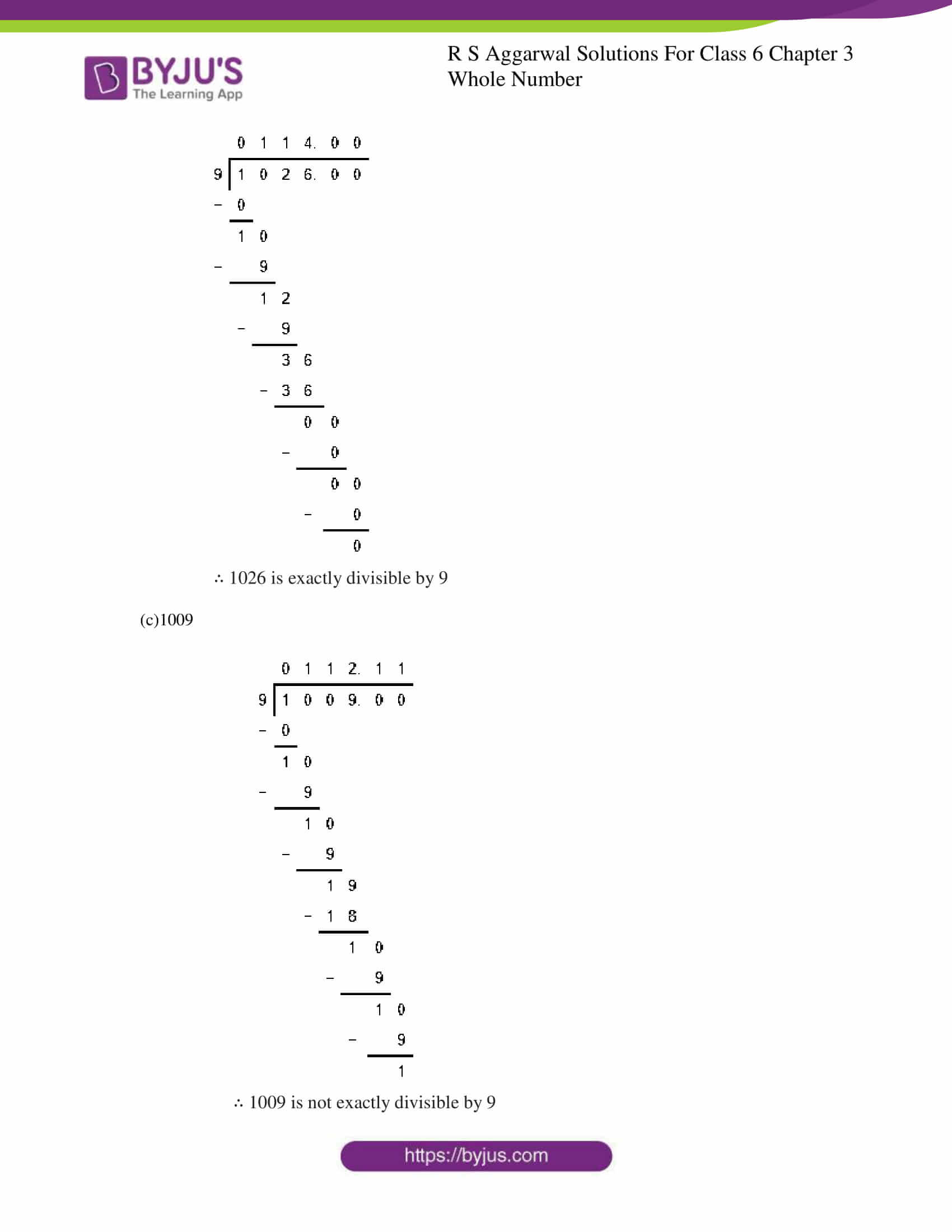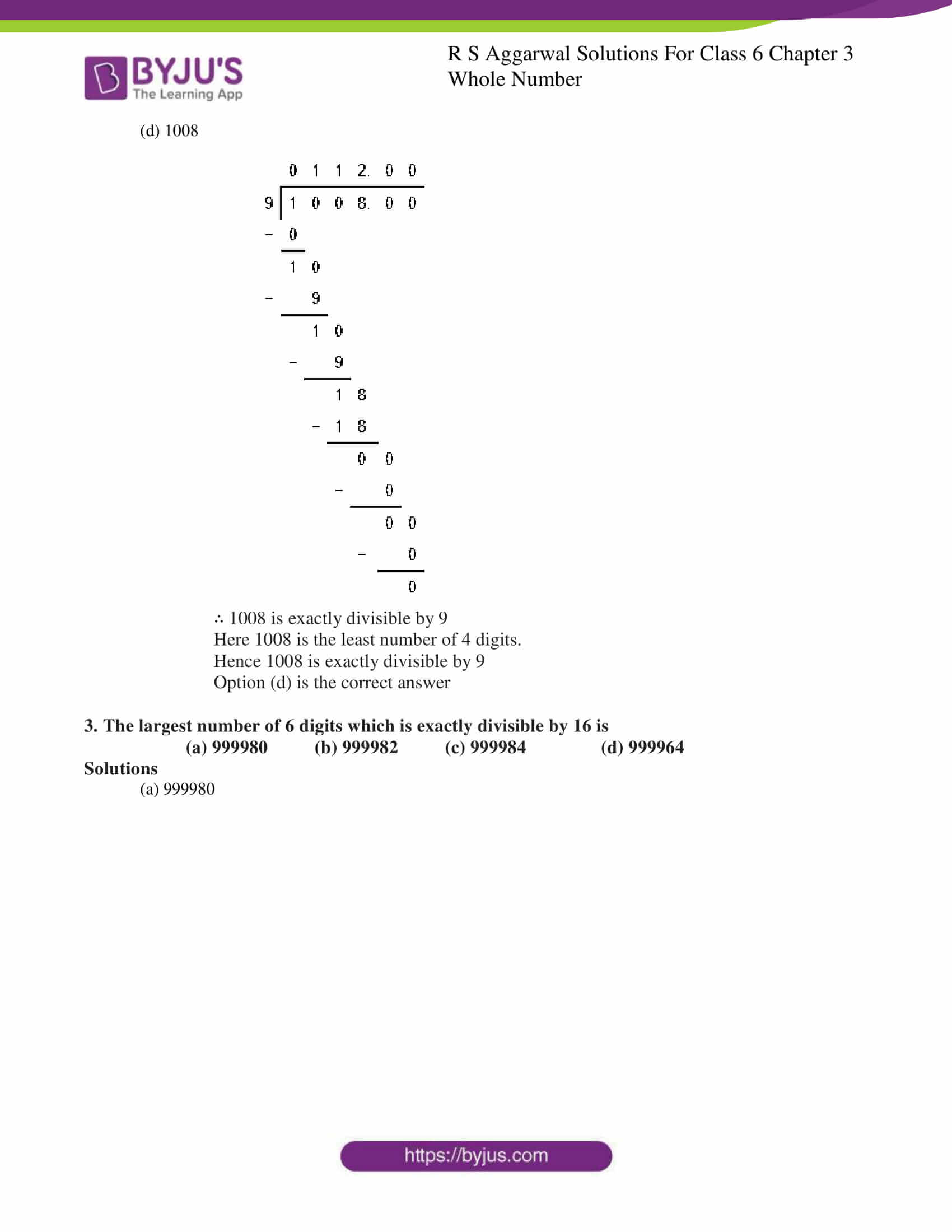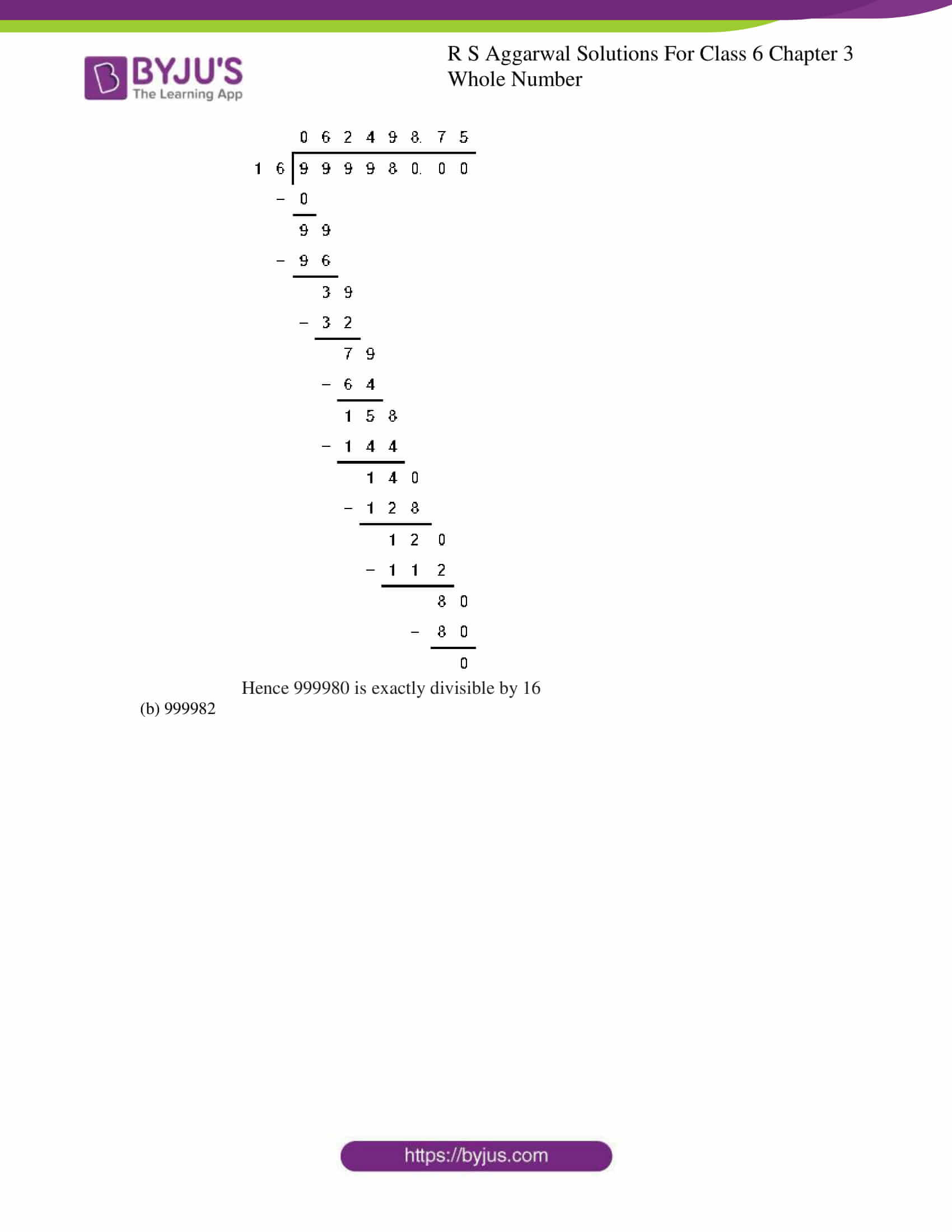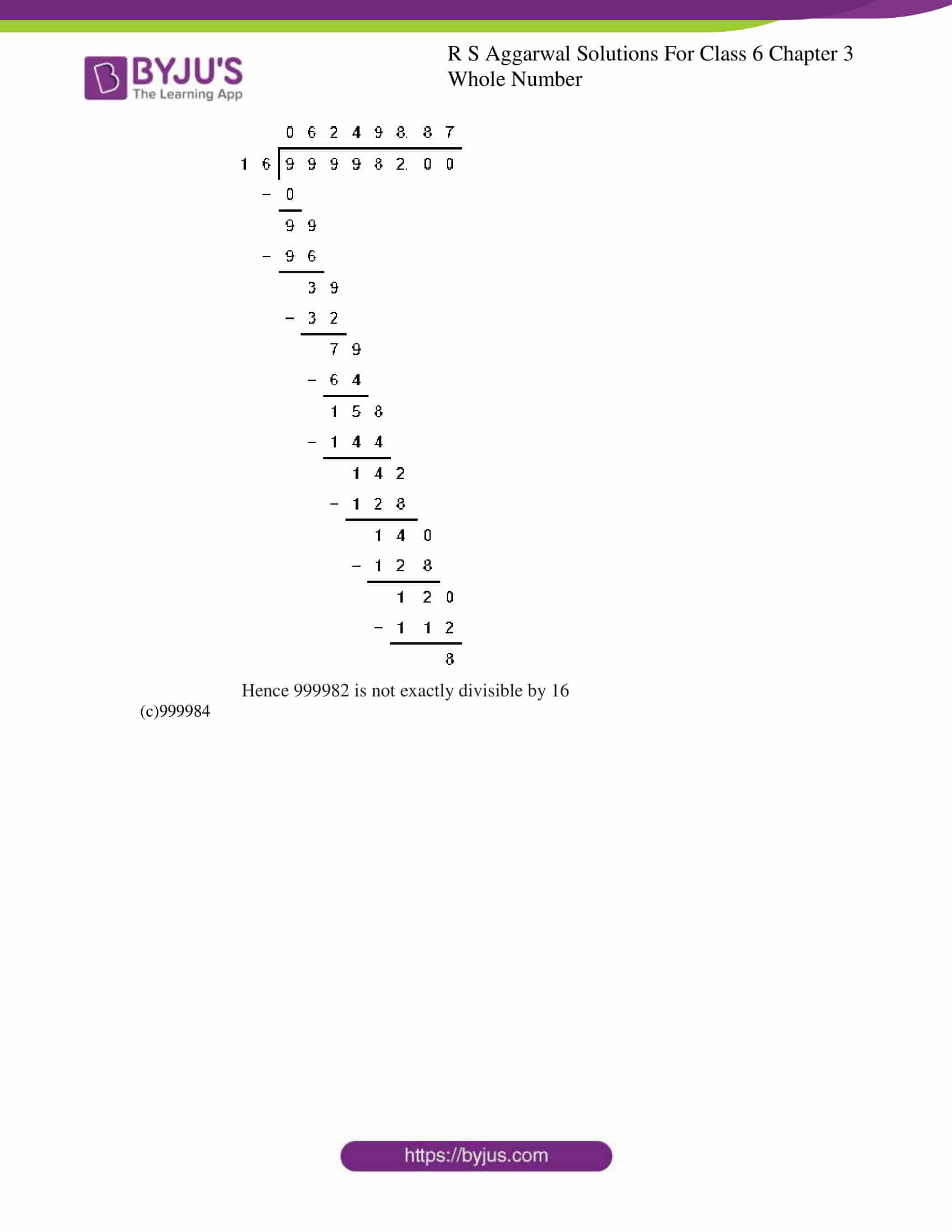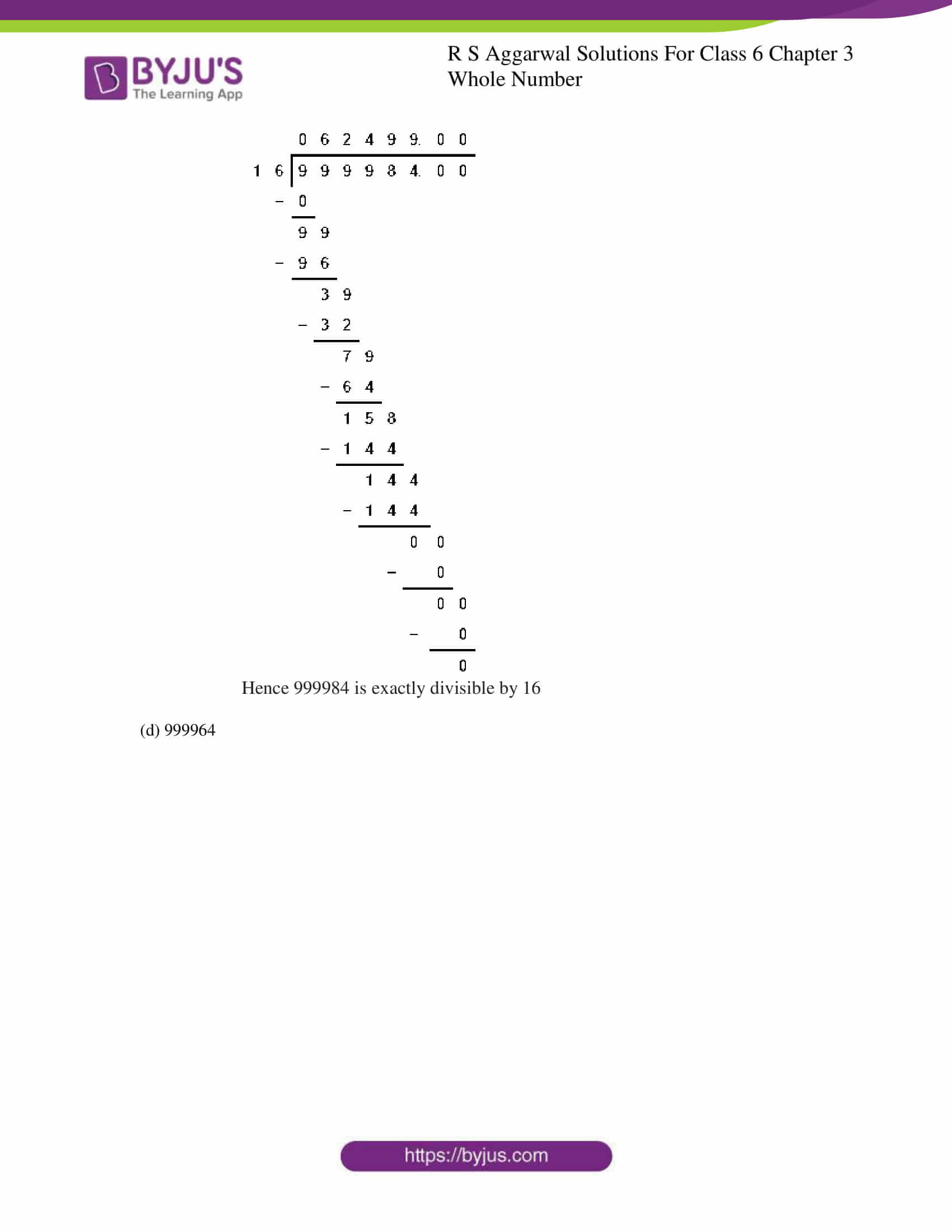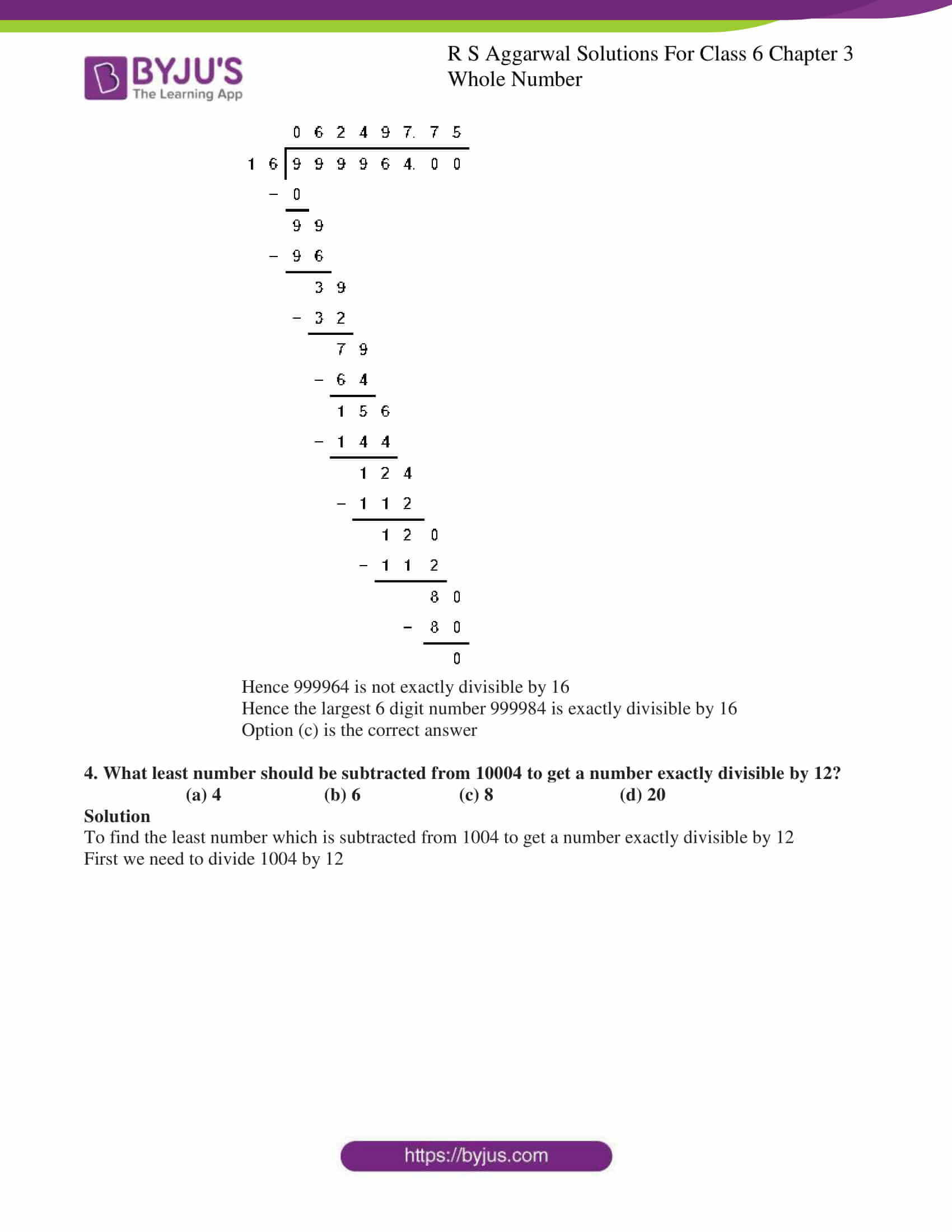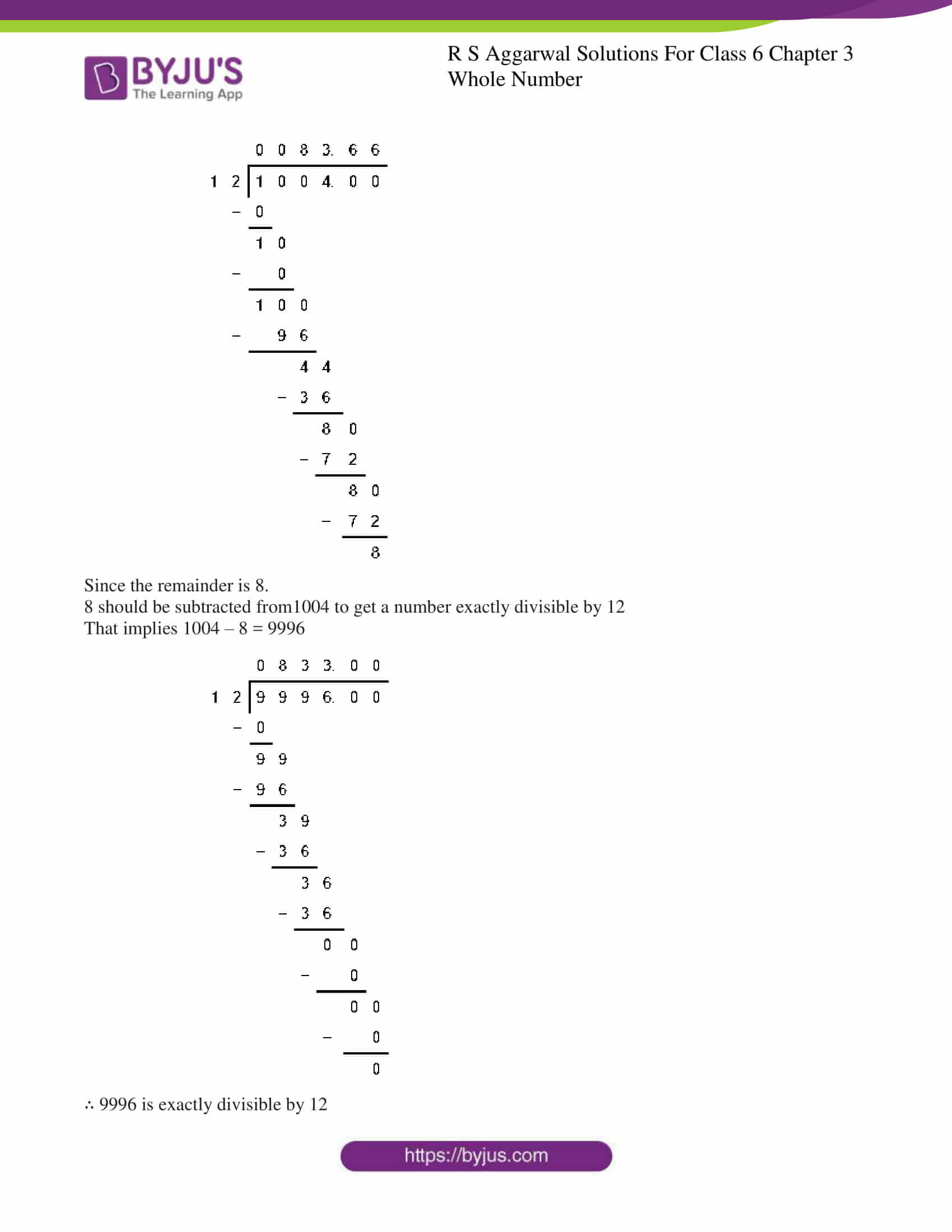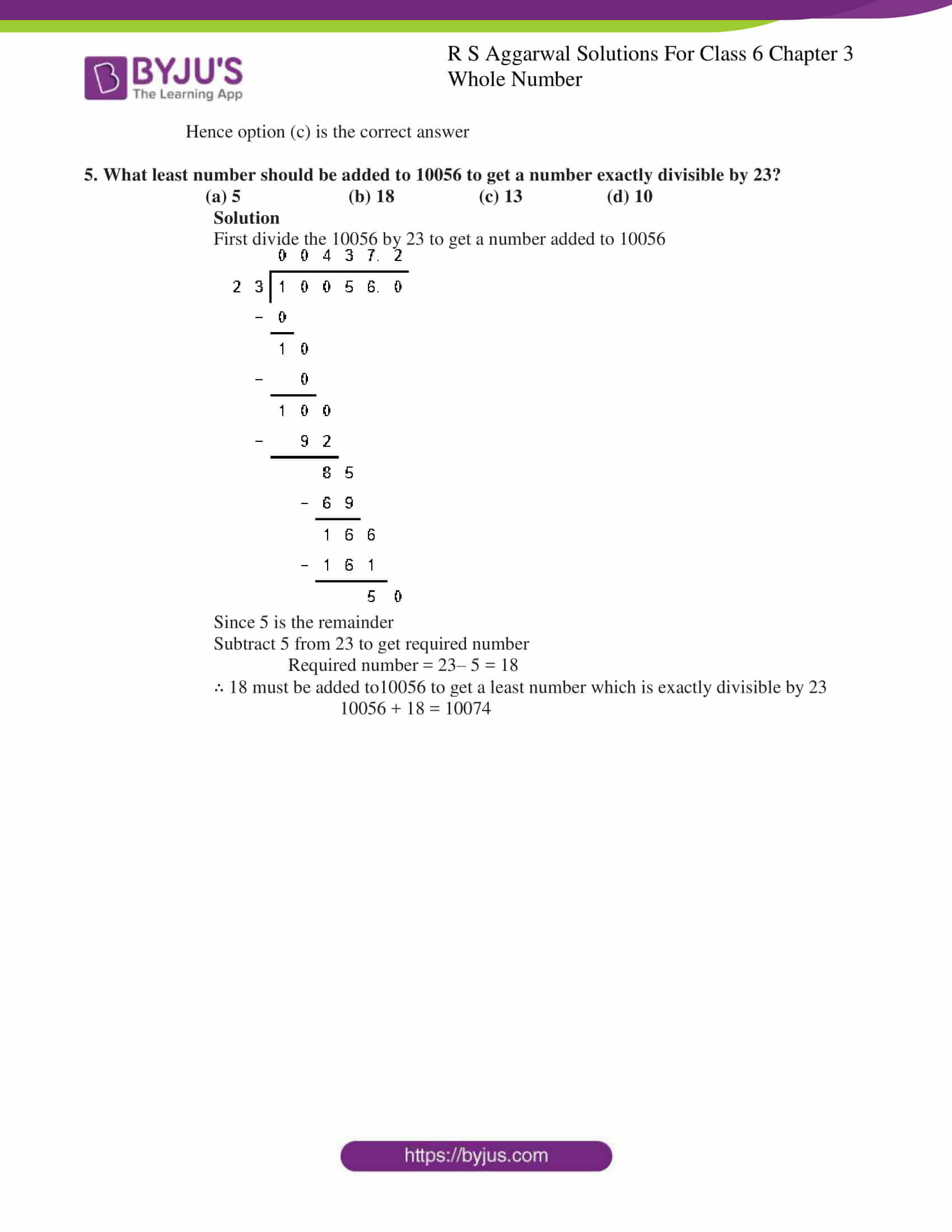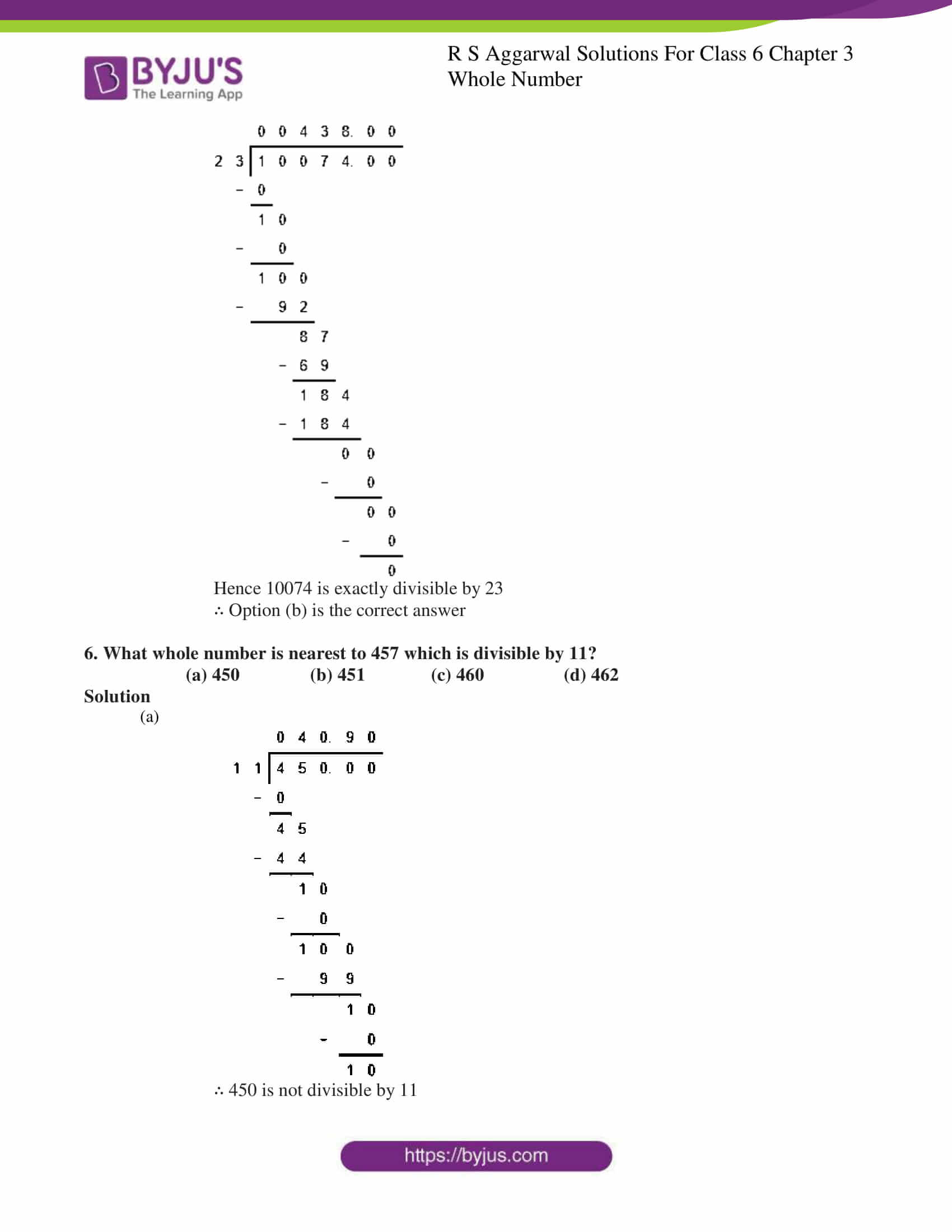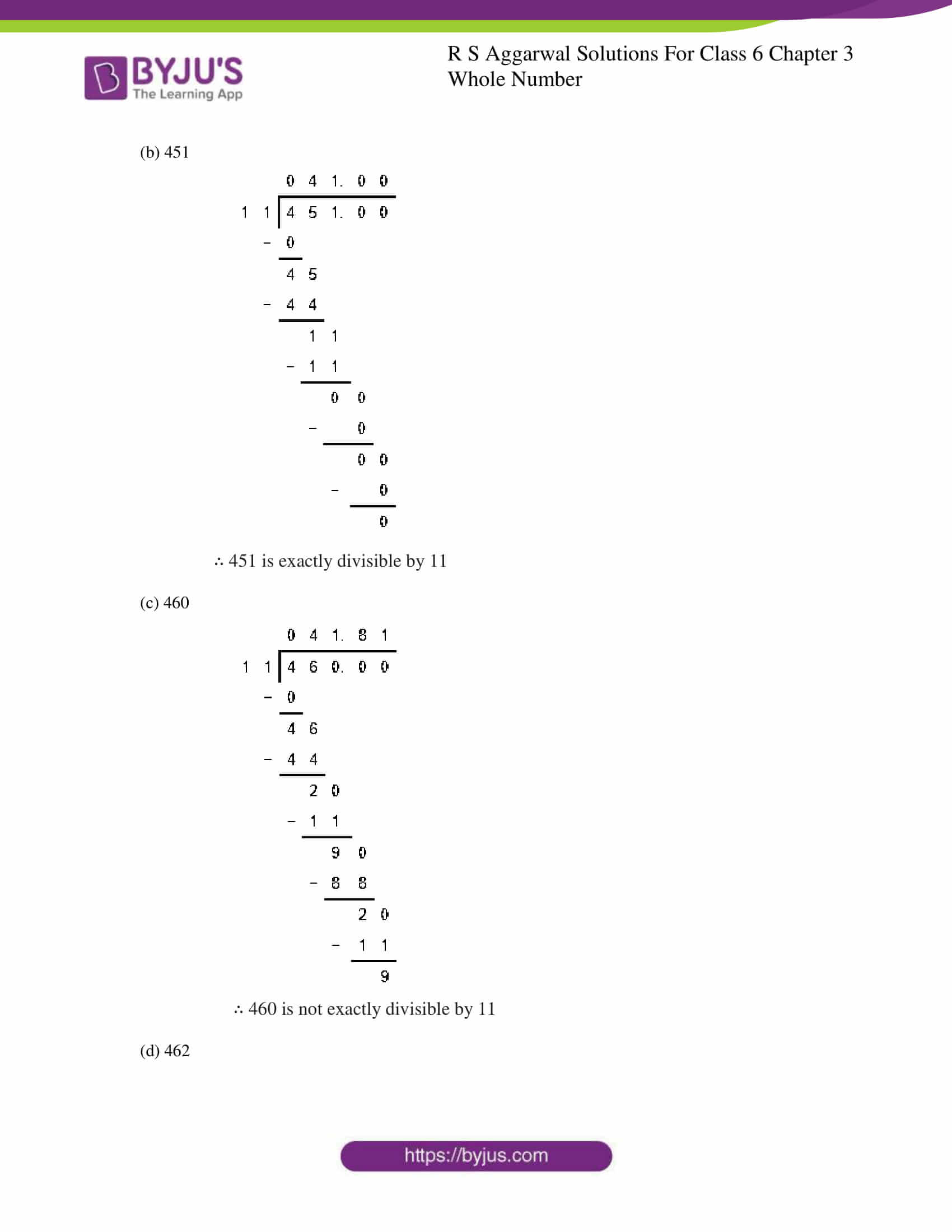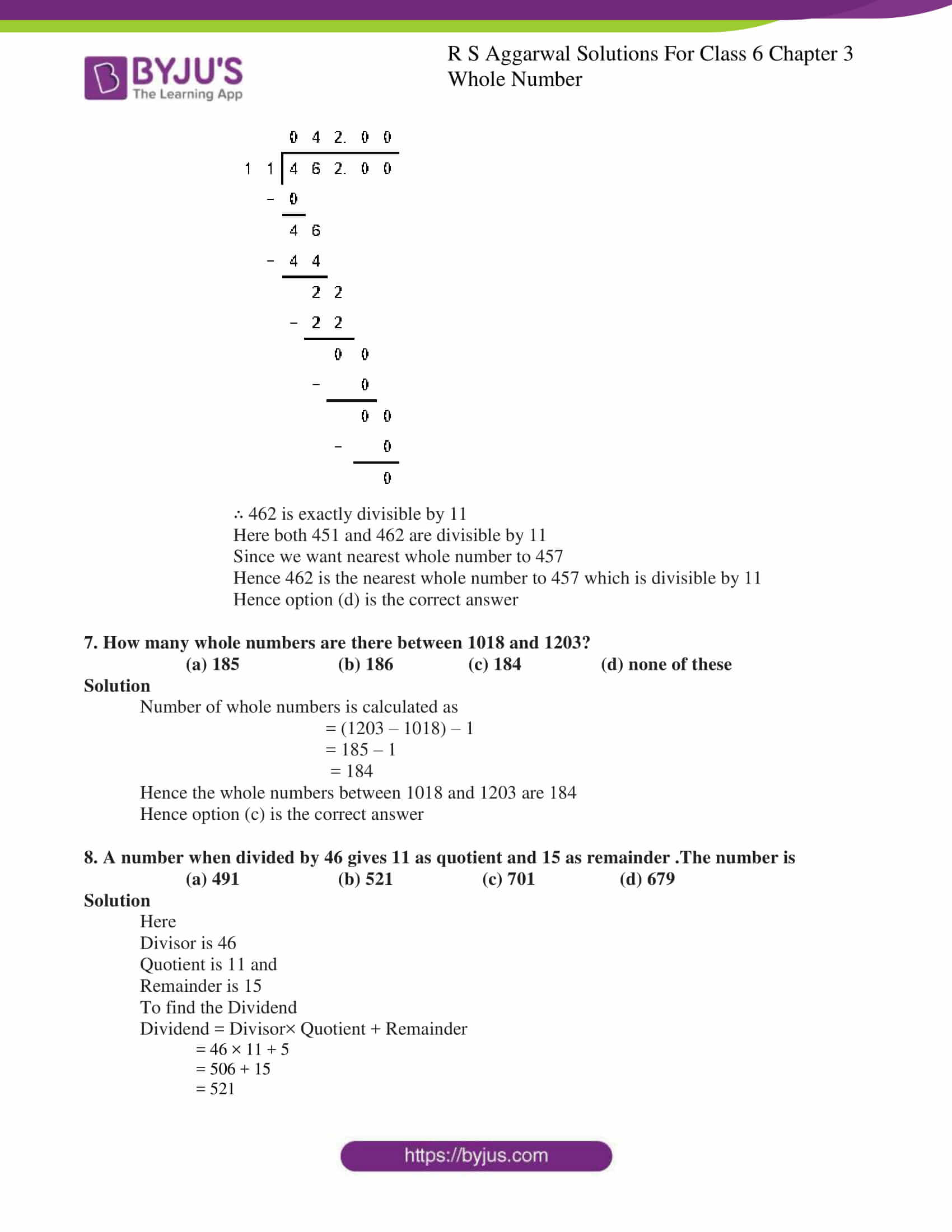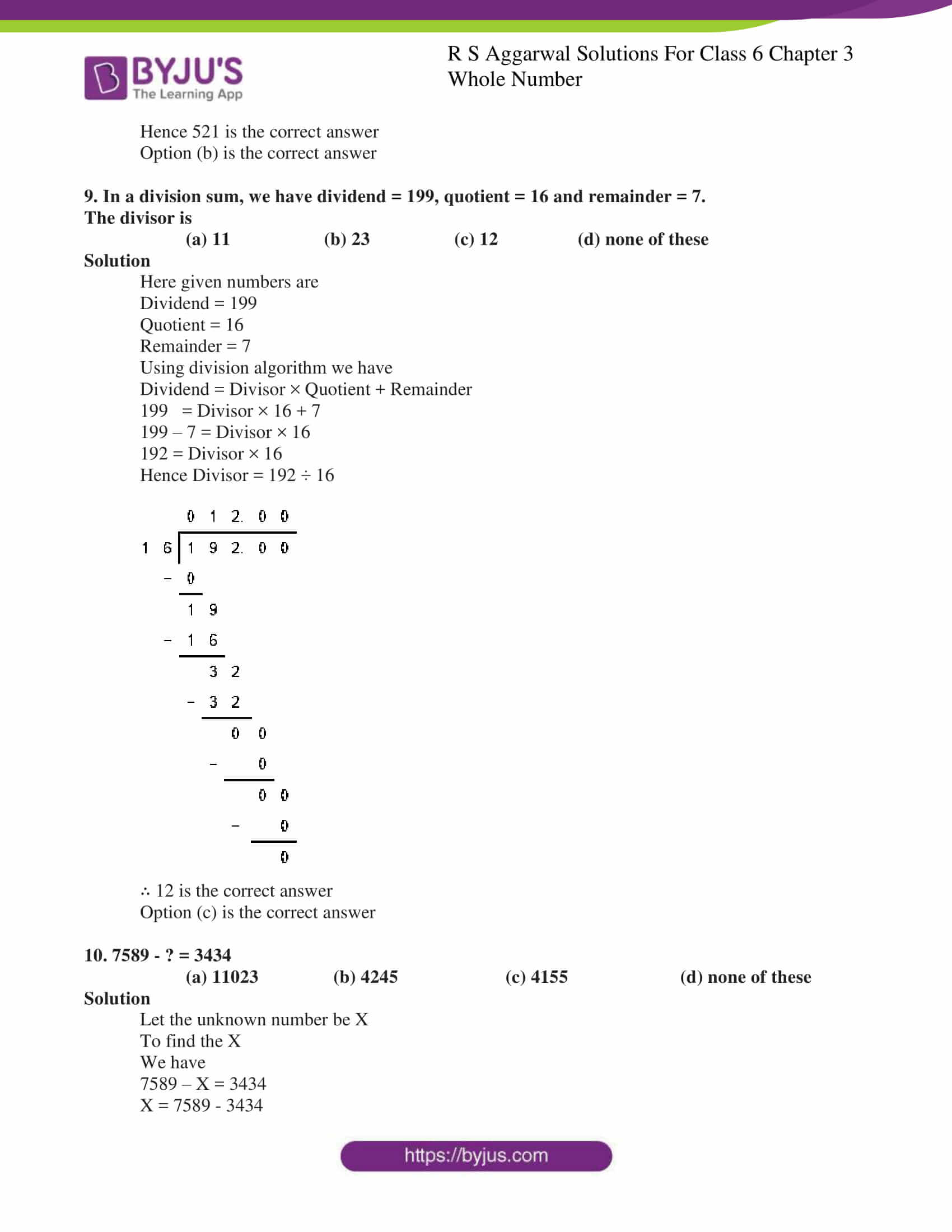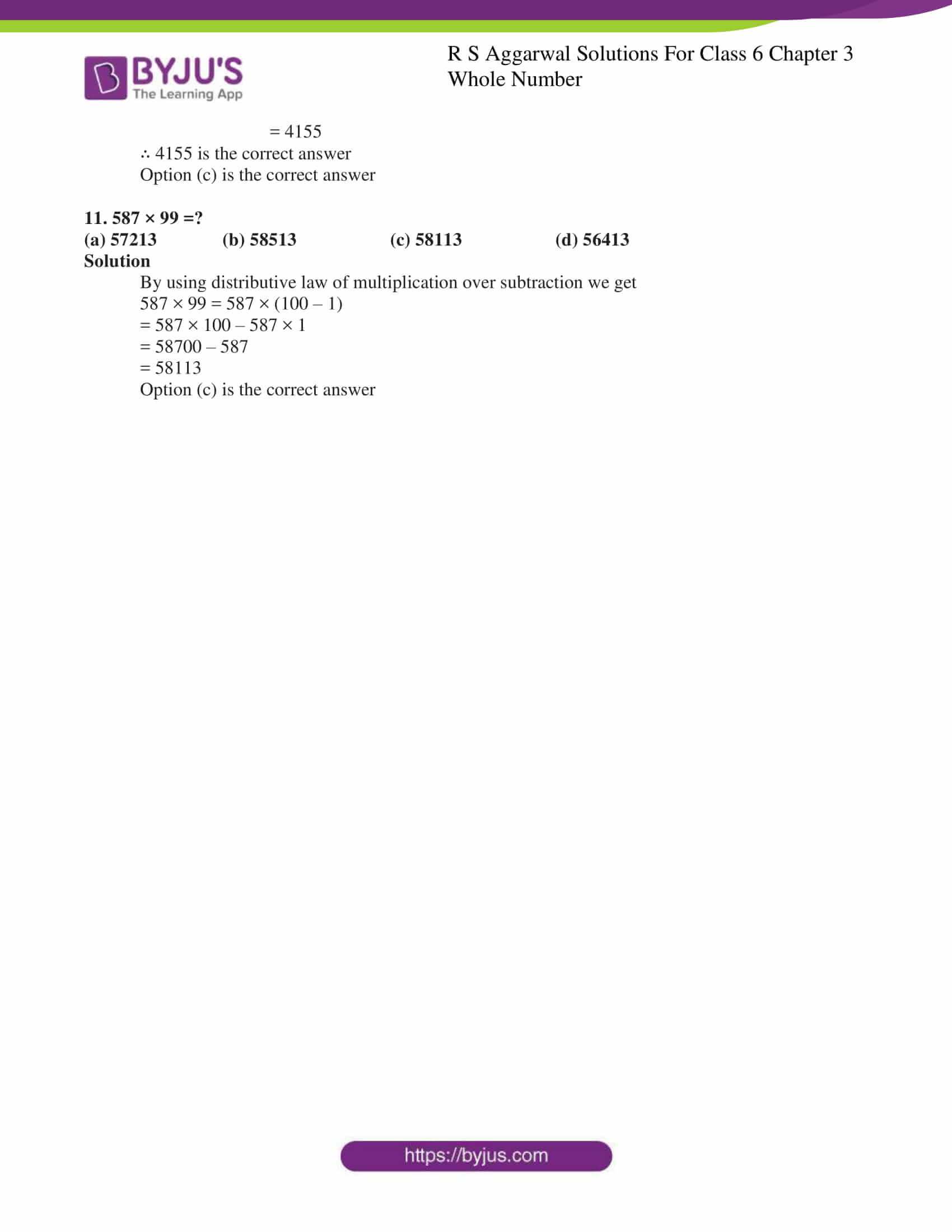### Access answers to Maths RS Aggarwal Solutions for Class 6 Chapter 3 Whole Numbers Exercise 3F

OBJECTIVE QUESTIONS

1. The smallest whole number is

(a) 1 (b) 0 (c) 2 (d) none of these

Solution

The smallest whole number is 0

Option is (b) is the correct answer

2. The least number of 4 digits which is exactly divisible by 9 is

(a) 1018 (b) 1026 (c) 1009 (d) 1008

Solution

(a) 1018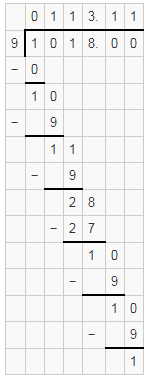∴ 1018 is not exactly divisible by 9

(b) 1026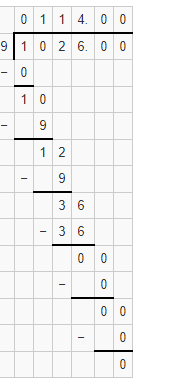∴ 1026 is exactly divisible by 9

(c)1009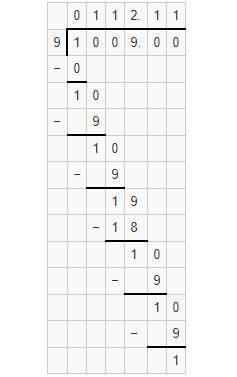∴ 1009 is not exactly divisible by 9

(d) 1008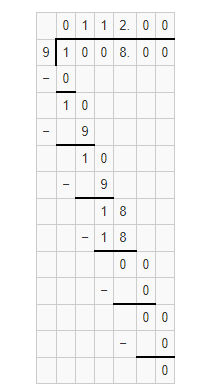∴ 1008 is exactly divisible by 9

Here 1008 is the least number of 4 digits.

Hence 1008 is exactly divisible by 9

Option (d) is the correct answer

3. The largest number of 6 digits which is exactly divisible by 16 is

(a) 999980 (b) 999982 (c) 999984 (d) 999964

Solutions

(a) 999980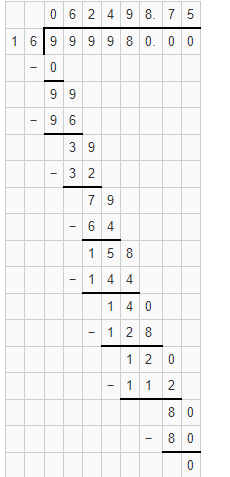Hence 999980 is exactly divisible by 16

(b) 999982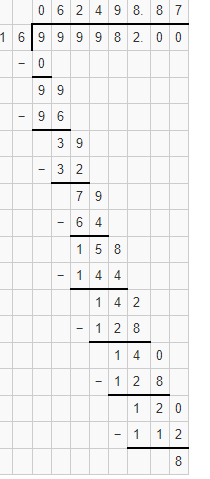Hence 999982 is not exactly divisible by 16

(c)999984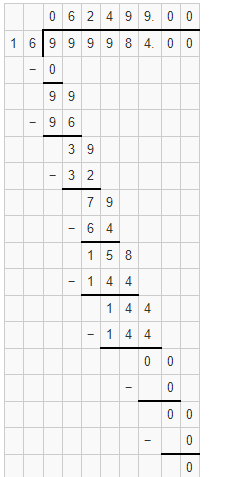Hence 999984 is exactly divisible by 16

(d) 999964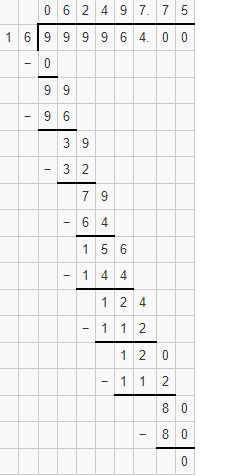Hence 999964 is not exactly divisible by 16

Hence the largest 6 digit number 999984 is exactly divisible by 16

Option (c) is the correct answer

4. What least number should be subtracted from 10004 to get a number exactly divisible by 12?

(a) 4 (b) 6 (c) 8 (d) 20

Solution

To find the least number which is subtracted from 1004 to get a number exactly divisible by 12

First we need to divide 1004 by 12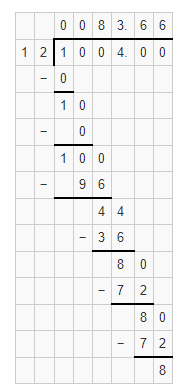Since the remainder is 8.

8 should be subtracted from1004 to get a number exactly divisible by 12

That implies 1004 – 8 = 9996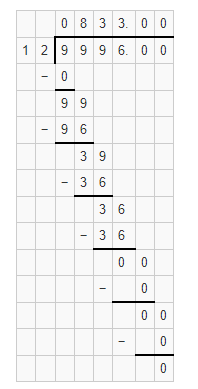∴ 9996 is exactly divisible by 12

Hence option (c) is the correct answer

5. What least number should be added to 10056 to get a number exactly divisible by 23?

(a) 5 (b) 18 (c) 13 (d) 10

Solution

First divide the 10056 by 23 to get a number added to 10056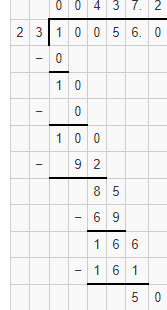Since 5 is the remainder

Subtract 5 from 23 to get required number

Required number = 23– 5 = 18

∴ 18 must be added to10056 to get a least number which is exactly divisible by 23

10056 + 18 = 10074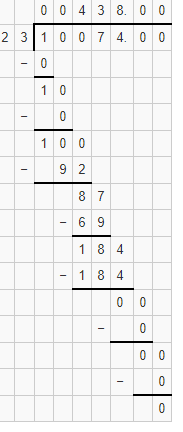Hence 10074 is exactly divisible by 23

∴ Option (b) is the correct answer

6. What whole number is nearest to 457 which is divisible by 11?

(a) 450 (b) 451 (c) 460 (d) 462

Solution

(a)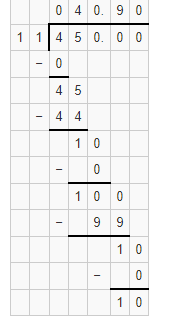∴ 450 is not divisible by 11

(b) 451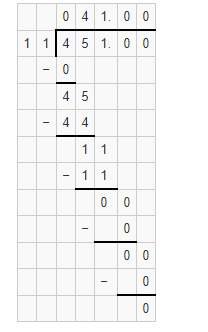∴ 451 is exactly divisible by 11

(c) 460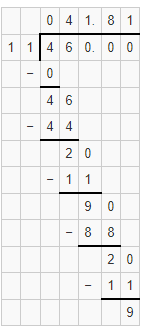∴ 460 is not exactly divisible by 11

(d) 462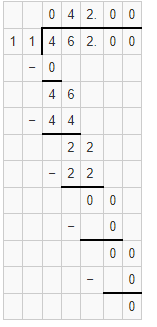∴ 462 is exactly divisible by 11

Here both 451 and 462 are divisible by 11

Since we want nearest whole number to 457

Hence 462 is the nearest whole number to 457 which is divisible by 11

Hence option (d) is the correct answer

7. How many whole numbers are there between 1018 and 1203?

(a) 185 (b) 186 (c) 184 (d) none of these

Solution

Number of whole numbers is calculated as

= (1203 – 1018) – 1

= 185 – 1

= 184

Hence the whole numbers between 1018 and 1203 are 184

Hence option (c) is the correct answer

8. A number when divided by 46 gives 11 as quotient and 15 as remainder .The number is

(a) 491 (b) 521 (c) 701 (d) 679

Solution

Here

Divisor is 46

Quotient is 11 and

Remainder is 15

To find the Dividend

Dividend = Divisor× Quotient + Remainder

= 46 × 11 + 5

= 506 + 15

= 521

Hence 521 is the correct answer

Option (b) is the correct answer

9. In a division sum, we have dividend = 199, quotient = 16 and remainder = 7.

The divisor is

(a) 11 (b) 23 (c) 12 (d) none of these

Solution

Here given numbers are

Dividend = 199

Quotient = 16

Remainder = 7

Using division algorithm we have

Dividend = Divisor × Quotient + Remainder

199 = Divisor × 16 + 7

199 – 7 = Divisor × 16

192 = Divisor × 16

Hence Divisor = 192 ÷ 16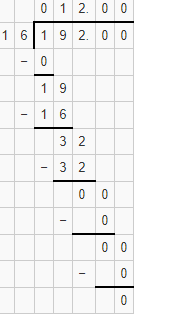∴ 12 is the correct answer

Option (c) is the correct answer

10. 7589 – ? = 3434

(a) 11023 (b) 4245 (c) 4155 (d) none of these

Solution

Let the unknown number be X

To find the X

We have

7589 – X = 3434

X = 7589 – 3434

= 4155

∴ 4155 is the correct answer

Option (c) is the correct answer

11. 587 × 99 =?

(a) 57213 (b) 58513 (c) 58113 (d) 56413

Solution

By using distributive law of multiplication over subtraction we get

587 × 99 = 587 × (100 – 1)

= 587 × 100 – 587 × 1

= 58700 – 587

= 58113

Option (c) is the correct answer